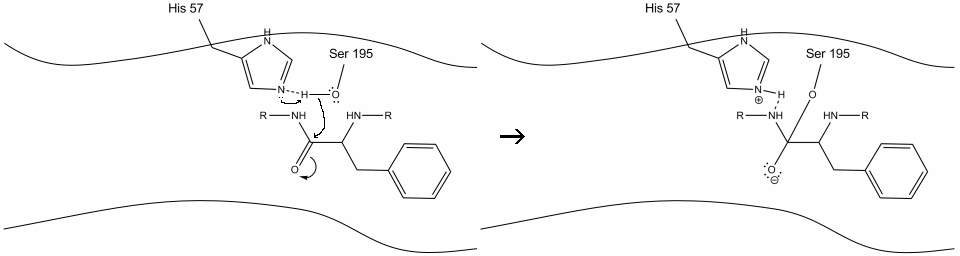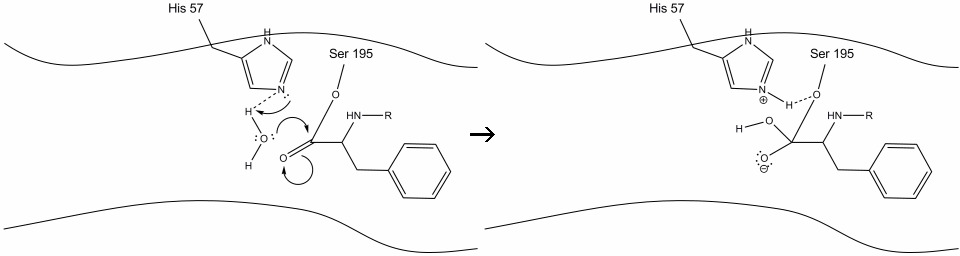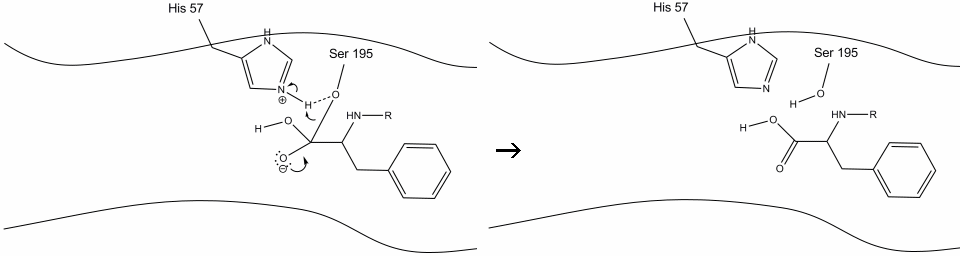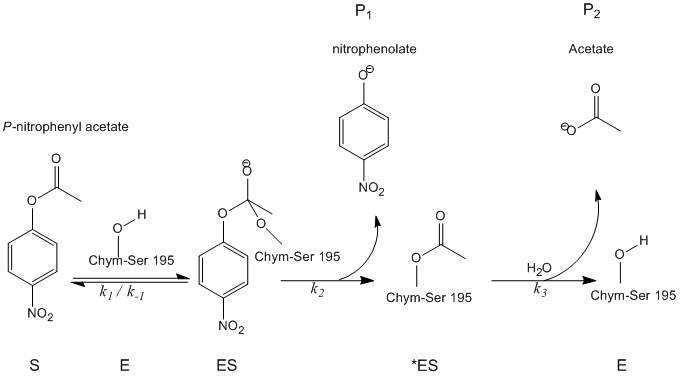# 9.3: Chymotrypsin- A Case Study

$$\newcommand{\vecs}{\overset { \rightharpoonup} {\mathbf{#1}} }$$ $$\newcommand{\vecd}{\overset{-\!-\!\rightharpoonup}{\vphantom{a}\smash {#1}}}$$$$\newcommand{\id}{\mathrm{id}}$$ $$\newcommand{\Span}{\mathrm{span}}$$ $$\newcommand{\kernel}{\mathrm{null}\,}$$ $$\newcommand{\range}{\mathrm{range}\,}$$ $$\newcommand{\RealPart}{\mathrm{Re}}$$ $$\newcommand{\ImaginaryPart}{\mathrm{Im}}$$ $$\newcommand{\Argument}{\mathrm{Arg}}$$ $$\newcommand{\norm}{\| #1 \|}$$ $$\newcommand{\inner}{\langle #1, #2 \rangle}$$ $$\newcommand{\Span}{\mathrm{span}}$$ $$\newcommand{\id}{\mathrm{id}}$$ $$\newcommand{\Span}{\mathrm{span}}$$ $$\newcommand{\kernel}{\mathrm{null}\,}$$ $$\newcommand{\range}{\mathrm{range}\,}$$ $$\newcommand{\RealPart}{\mathrm{Re}}$$ $$\newcommand{\ImaginaryPart}{\mathrm{Im}}$$ $$\newcommand{\Argument}{\mathrm{Arg}}$$ $$\newcommand{\norm}{\| #1 \|}$$ $$\newcommand{\inner}{\langle #1, #2 \rangle}$$ $$\newcommand{\Span}{\mathrm{span}}$$$$\newcommand{\AA}{\unicode[.8,0]{x212B}}$$

Chymotrypsin is a digestive enzyme belonging to a super family of enzymes called serine proteases. It uses an active serine residue to perform hydrolysis on the C-terminus of the aromatic amino acids of other proteins. Chymotrypsin is a protease enzyme that cleaves on the C-terminal phenylalanine (F), tryptophan (W), and tyrosine (Y) on peptide chains. It shows specificity for aromatic amino acids because of its hydrophobic pocket.

## Introduction

Chymotrypsin is one of the most studied enzymes due to its two phase kinetics: pre-steady-state and steady state. The study of these two kinetic states gives evidence of the "Ping-Pong" mechanism, the formation of covalent complexes leading to covalent hydrolysis reactions, and the rate of the catalyzed reactions. Synthesis of chymotrypsin occurs primarily in the pancreas. Instead of the active form, however, it is produced as an inactive zymogen called chymotrypsinogen to prevent its protease activity from digesting the pancreas. Upon secretion into the lumen of the small intestine, it is converted to its active form by another enzyme called trypsin. This dependence of a different enzyme for the activation of a protease is a common way for the body to prevent the digestion of organs and other harmful enzymatic side-effects.

Chymotrypsin operates through a general mechanism known as the ping-pong mechanism (Figure $$\PageIndex{1}$$) whereby the enzyme reacts with a substrate to form an enzyme intermediate. This intermediate has different properties than the initial enzyme, so to regenerate the initial enzymatic activity, it must react with a secondary substrate. This process is illustrated below:Figure $$\PageIndex{1}$$: Generic Ping-Pong Mechanism

More specifically, chymotrypsin operates through a particular type of ping-pong mechanism called covalent hydrolysis. This means that the enzyme first forms a covalent bond with the target substrate, displacing the more stable moiety into solution. This enzyme-substrate complex is called the enzyme intermediate. The intermediate then reacts with water, which displaces the remaining part of the initial substrate and reforms the initial enzyme.Chymotrypsin, like most enzymes, is specific in the types of substrates with which it reacts. As a protease, it cleaves polypeptides, and its inherent specificity allows it to act only on the carboxy-terminal of aromatic residues. It is a somewhat complicated mechanism, and is best explained in a series of steps.

Step 1: The target enters the active site of chymotrypsin, and it is held there by hydrophobic interactions between exposed non-polar groups of enzyme residues and the non-polar aromatic side-chain of the substrate. It is important to note the hydrogen bond between the Schiff nitrogen on histidine-57 and the oxygen side-chain of serine-195.Step 2: Aided by the histidine-serine hydrogen bonding, the hydroxyl group on serine-195 performs a nucleophilic attack on the carbonyl carbon of an aromatic amino acid while simultaneously transferring the hydroxyl hydrogen to the histidine Schiff nitrogen. This attack pushes the pi carbonyl electrons onto the carbonyl oxygen, forming a short-lived intermediate consisting of a c-terminal carbon with four single bonds: an oxygen anion, the beta-carbon of the aromatic amino acid, the n-terminus of the subsequent amino acid of the substrate protein, and the serine-195 side-chain oxygen.Step 3: This intermediate is short-lived, as the oxyanion electrons reform the pi bond with the c-terminus of the aromatic amino acid. The bond between the carboxy-terminus of the aromatic amino acid and the n-terminus of the subsequent residue is cleaved, and its electrons are used to extract the hydrogen of the protonated Schiff nitrogen on histidine-57. The bonds between the carbonyl carbon and the serine-195 oxygen remain in an ester configuration. This is called the acyl-enzyme intermediate. The c-terminal side of the polypeptide is now free to dissociate from the active site of the enzyme.Step 4: Water molecules are now able to enter and bind to active site through hydrogen bonding between the hydrogen atoms of water and the histidine-57 Schiff nitrogen.Step 5: The water oxygen now makes a nucleophilic attack on the carbonyl carbon of the acyl-enzyme intermediate, pushing the carbonyl’s pi electrons onto the carbonyl carbon as histidine-57 extracts one proton from water. This forms another quaternary carbon covalently bonded with serine, a hydroxyl, an oxyanion, and the aromatic amino acid. The proton on the recently protonated histidine-57 is now able to make a hydrogen bond with the serine oxygen.Step 6: The oxyanion electrons reform the carbonyl pi bond, cleaving the bond between the carbonyl carbon and the serine hydroxyl. The electrons in this bond are used by the serine oxygen to deprotonate the histidine Schiff nitrogen and reform the original enzyme. The substrate no longer has affinity for the active site, and it soon dissociates from the complex.## Kinetics

Experiments were conducted in 1953 by B.S. Hartley and B.A. Kilby to investigate the kinetics of chymotrypsin-catalyzed hydrolysis. Instead of using a poly-peptide chain as a substrate, they used a nitro-phenyl ester, p-nitrophenyl acetate, that resembles an aromatic amino acid. Hydrolysis of this compound by chymotrypsin at the carbonyl group yields acetate and nitrophenolate, the latter of which absorbs near 400 nm light and its concentration can thus be measured by spectrophotometry (Figure $$\PageIndex{2}$$).Figure $$\PageIndex{2}$$: Catalytic activity of he hydrolysis of p-nitropenolate under

Spectrophotometric analysis of chymotrypsin acting on nitrophenylacetate showed that nitrophenolate was produced at a rate independent of substrate concentration, proving that the only factor contributing to the rate of product formation is the concentration of enzyme; this is typical for enzyme-substrate kinetics. However, when the slope of the 0-order absorbance plot was traced back to the starting point (time = 0), it was found that the initial concentration of nitrophenolate was not 0. In fact, it showed a 1:1 stoichiometric ratio with the amount of chymotrypsin used in the assay. This can only be explained by the fact that hydrolysis by chymotrypsin is biphasic in nature, meaning that it proceeds in two distinct steps.

1. The first step, which describes the initial burst of nitrophenolate seen in Hartley and Kilby’s absorbance plot, is the fastest. The attack of the nitrophenyl acetate substrate by chymotrypsin immediately cleaves the nitrophenolate moiety and leaves the acetate group attached to chymotrypsin, rendering the enzyme inactive.
2. The second step has been deduced to involve the hydrolysis of the acetate group from the inactivated chymotrypsin to regenerate the original enzyme.

To analytically determine the rate of catalysis, all substrates, products, and intermediates must be defined. Refer to the figure below:Using these abbreviations, kinetic analysis becomes less cumbersome.

1. The initial amount of enzyme can be represented as the sum of the free enzyme, the bound enzyme, and the inactive intermediate.

$[E]_o = [ES] + [^*ES] + [E]$

2. Assuming the initial step is the faster than the subsequent steps, the rate of nitrophenolate production can be described as:

$\dfrac{d[P_1]}{dt}=k_2[ES]$

3. Likewise, the rate of acetate formation can be represented by the equation:

$\dfrac{d[P_2]}{dt}=k_3[^*ES]$

4. Therefore, the net change in concentration of the inactive intermediate can be deduced:

$\dfrac{d[*ES]}{dt}=k_2[ES]-k_3[^*ES]$

5. The last inference that can be made from analysis of the measured kinetics data (Figure $$\PageIndex{2}$$) is that the first step of the reaction equilibrates rapidly, and thus the change in bound substrate can be described in the following equation. This is a principal tenet in analyzing the kinetics of chymotrypsin and is a ubiquitous mechanism in biological enzyme catalysis.

$\dfrac{d[ES]}{dt}=k_1[E][S]-k_{-1}[ES]=0$

6. Where:

$\dfrac{k_{-1}}{k_1} = K_s = \dfrac{[E][S]}{[ES]}$

7. Combining all of these quantities, we can deduce the catalytic rate constant as:

$k_{cat} = \dfrac{k_2k_3}{k_2+k_3}$

8. In ester hydrolysis, $$k_3 >> k_2$$, so the resultant catalytic rate constant simplifies to:

$k_{cat}=k_2$

which is in agreement with the observed zeroth-order kinetics of Figure $$\PageIndex{2}$$.

 k2 0.37 ± 0.11 s-1 k3 (1.3 ± 0.02) × 10-4 s-1 Ks (1.6 ± 0.5) × 10-3 kcat 1.3 × 10-4 s-1 KM 5.6 × 10-7
##### Exercise $$\PageIndex{1}$$

Speculate on how the catalytic rate constant can be determined from the spectrophotogram.

The catalytic rate constant can be deduced from the graph by simply determining the slope of the line where the reaction demonstrates 0-order kinetics (the linear part)

##### Exercise $$\PageIndex{2}$$

How can product be consistently produced if the rate of change of the ES complex is 0?

This is pre-equilibrium kinetics in action. The ES complex is formed from E and S at a faster rate than any other step in the reaction. As soon as ES is converted to *ES, another mole of ES is produced from an infinite supply of E + S. This means that the amount of ES and E + S is constantly at equilibrium, and thus the change of either with respect to time is 0.

##### Exercise $$\PageIndex{3}$$

How would the rate of product formation change if:

1. the substrate concentration was doubled.
2. the enzyme concentration was doubled.
3. The reaction was carried out in mono-deuterated water instead of H2O (comment qualitatively).
1. No change.
2. Two fold increase.
3. Since water is involved in the final, slowest step of the mechanism, deuterating the water would decrease the rate of the overall reaction from 5 to 30-fold.
##### Exercise $$\PageIndex{4}$$

Explain the role of hydrogen bonding in protein hydrolysis catalyzed by chymotrypsin.

Initially, hydrogen bonding between the enzymes histidine and serine side chains weakens the bond of serine’s O-H. This allows for a facilitated nucleophilic attack of the hydroxyl Oxygen on the substrates carbonyl group. Conversely, in the final step of the reaction, the bound serine oxygen forms a hydrogen bond with a protonated histidine, which allows for easier cleavage from the substrate.

##### Exercise $$\PageIndex{5}$$

What would the spectrophotogram look like if the reaction proceeded via a steady-state mechanism instead of pre-equilibrium.

The graph would show similar 0-order kinetics, but the line would intercept the Y-axis at an absorbance of 0 instead of the 1:1 mole ratio of nitrophenolate to enzyme.

## References

1. Chang, Raymond. Physical Chemistry for the Biosciences. Sansalito, CA: University Science, 2005.
2. Segel, Irwin H., Enzyme Kinetics: Behavior and Analysis of Rapid Equilibrium and Steady-State Enzyme Systems (Wiley Classics Library)
3. Garret, Reginald. Grishman, Charles. Biochemistry. University of Virginia, 2007
4. Whitaker, John., Princles of Enzymology for the Food Sciences. University of California, Davis. 1994
5. “Chymotrypsin Mechanism” viewable at: www.sumanasinc.com/webcontent...motrypsin.html
6. Sumanas Inc, viewed on February 25th, 2009.
7. M.L. Bender, F.J. Kezdy and F.C. Wedler, J. Chem. Educ. 44, 84 (1967)

9.3: Chymotrypsin- A Case Study is shared under a not declared license and was authored, remixed, and/or curated by LibreTexts.